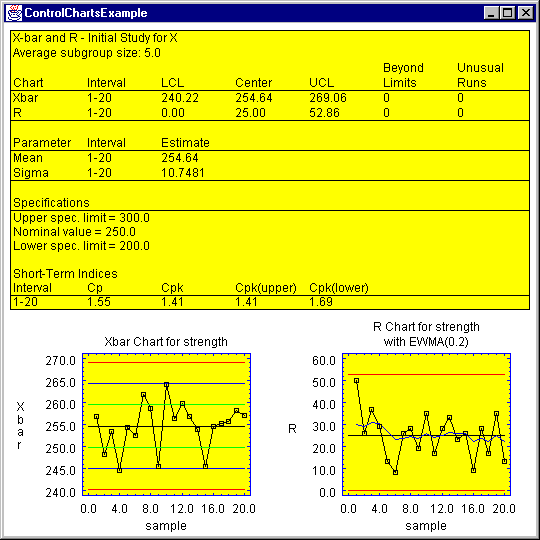## JavaBean Components for Statistical Analysis

### Contents

Overview
Types of STATBEANS
Installation
DataSource StatBeans
Calculation StatBeans
Tabular StatBeans
Graphical StatBeans
Developing an Application
Notes
Examples

### Overview

STATBEANS® is a collection of Java Beans which implement many commonly used statistical procedures. They are designed to be embedded in user-written applications or placed on web pages. Because of their structure as a component library, they may be easily manipulated in various visual development environments. Users have a choice of accessing STATBEANS as JavaBeans or as ActiveX components using the JavaBeans-ActiveX bridge.

### Types of STATBEANS

There are four basic types of StatBeans: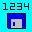DataSource StatBeans - these beans maintain a rectangular data table which other StatBeans access to retrieve data for analysis. DataSource StatBeans are provided for reading data from local text files, for reading data over the Internet or local intranets, for accessing databases via JDBC, and for maintaining data generated by user programs.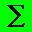Calculation StatBeans - these are non-visible beans which perform statistical calculations. They may be called by user programs to calculate statistics. They are also accessed by the tabular and graphical StatBeans.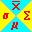Tabular StatBeans - these StatBeans perform statistical calculations and display them in the form of tables.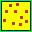Graphical StatBeans - these StatBeans perform statistical calculations and display them in the form of graphs. Users create applications by first adding one or more datasource StatBeans to their project, and then linking the other StatBeans to the datasource.

### Installation

STATBEANS consists of a collection of components which are packaged in a file called statbeans.jar. To install it:
1. Place the jar file in a new directory called statbeans.
2. Update the CLASSPATH environment variable to include statbeans\statbeans.jar.
3. Import the jar file into your application development tool, if desired.

There are a number of other useful files distributed with the package:

• Documentation files - the documentation for STATBEANS consists of a set of html files providing an overview of the system and describing each StatBean.
• Sample applications - sample java source files showing how each StatBean may be used. These examples demonstrate both how to use the StatBeans with data generated by an application and how to hook them to external data files and databases. Several sample data files are also included.
• ### DataSource StatBeans

DataSource StatBeans maintain rectangular data tables into which data is loaded for access by other types of StatBeans. Each project must contain at least one datasource StatBean. Five types of datasource StatBeans are provided:
• FileDataSource - reads data from file on a local disk.
• JdbcDataSource - retrieves data from a database via an SQL query using JDBC.
• ProgramDataSource - maintains a data table for holding data generated by a user-written Java program.
• UrlDataSource - reads data from a file located on the Internet or an intranet via its URL.
• Calculation Statbeans - these beans inherit the data from their parent DataSource and add other variables which they calculate (such as residuals from a regression).

### Calculation StatBeans

Calculation StatBeans compute statistics which may then be accessed by user programs or other StatBeans. They retrieve data from a datasource StatBean by specifying one or more column names. Each calculation StatBean is set up to listen for that datasource's dataChange event, which causes it to request data from the datasource and perform its calculations. Results may then be obtained from the calculation StatBean by invoking one of its methods.

The following calculation StatBeans are currently available:

• Anova - multifactor analysis of variance.
• Autocorrelations - calculates sample autocorrelations and partial autocorrelations for a time series.
• CapabilityAnalysis - compares data to process specification limits.
• ContingencyTableStats - calculates measures of association for rows and columns in contingency tables.
• ControlCharts - calculates control charts for variables and attributes.
• Correlations - estimates correlation coefficients between pairs of numeric variables.
• Crosstabulation - creates a contingency table for 2 categorical or numeric data variables.
• Distributions - computes probabilities and generates random numbers for 24 probability distributions.
• FitDistribution - fits distributions to a column of data, computes probabilities, and generates random numbers.
• GageRandR - estimates gage repeatability and reproducibility.
• HypothesisTests - performs hypothesis tests for means, medians, standard deviations, proportions, and rates.
• MultipleRegression - fits a regression model to relate Y to one or more predictor variables.
• NonlinearRegression - fits a non-linear regression model to relate Y to one or more predictor variables.
• Percentiles - calculates percentiles for a column of numeric data.
• Periodogram - calculates periodogram ordinates for a time series.
• PolynomialRegression - fits a polynomial model to relate Y and X.
• SampleStatistics - calculates sample statistics for two or more columns of data.
• SimpleRegression - fits a linear or curvilinear model to relate Y and X.
• Tabulation - tabulates categorical or numeric data.
• TimeSeriesAdjustments - applies various mathematical and other adjustments to a time series.
• TimeSeriesForecast - forecasts values of a time series.
• TimeSeriesSmoothing - applies different types of smoothers to a time series.
• ToleranceLimits - calculates normal tolerance limits for a column of numeric data.

### Tabular StatBeans

Tabular StatBeans compute statistics and display them in the form of tables. They usually retrieve their data and results from a Calculation StatBean, although simple Tabular StatBeans (such as DataDisplayTable) retrieve their data directly from a DataSource StatBean.

The following Tabular StatBeans are currently available:

### Graphical StatBeans

Graphical StatBeans compute statistics and display them in the form of graphs. They usually retrieve their data and results from a Calculation StatBean, although simple Graphical StatBeans (such as XYPlot) retrieve their data directly from a DataSource StatBean.

The following Graphical StatBeans are currently available:

### Developing an Application

To develop an application which uses STATBEANS, you can use a visual development tool such as Visual Cafe or Visual Age for Java, or you can manipulate the components directly. In most development tools, you begin by adding the statbeans.jar file to a component library, after which you can drop the components onto a design form.

To develop an application, several steps are then necessary:

• STEP 1: add a datasource Statbean to the project and set its properties. For example, to read a file, you would insert the FileDataSource bean into your project and set the fileName property to the name of the file you wanted to read. The following lines are needed:

import STATBEANS.FileDataSource;
fileDataSource1 = new FileDataSource();
fileDataSource1.setFileName("c:\\statbeans\\samples\\cardata.txt");
• STEP 2: add a Calculation Statbean to the project and set its properties. For example, to fit a straight line relating two columns of data in the datasource named "mpg" and "horsepower", you would insert the SimpleRegression bean into your project, and then set the XVariableName property and YVariableName property to the names of the columns to be analyzed. The following lines are needed:

import STATBEANS.SimpleRegression;
simpleRegression1 = new STATBEANS.SimpleRegression();
simpleRegression1.setYVariableName("mpg");
simpleRegression1.setXVariableName("weight");

• STEP 3: add one or more Tabular and Graphical Statbeans to the project and set its properties. For example, to display the results of Step 2, you would insert the SimpleRegressionTable and SimpleRegressionPlot beans into your project. The following lines are needed:

import STATBEANS.SimpleRegressionTable;
import STATBEANS.SimpleRegressionPlot;
simpleRegressionTable1 = new STATBEANS.SimpleRegressionTable();
simpleRegressionPlot1 = new STATBEANS.SimpleRegressionPlot();
simpleRegressionPlot1.setConfidenceLevel(99.0);
• STEP 4: connect the SimpleRegression bean to the FileDataSource bean. Also connect the SimpleRegressionTable and SimpleRegressionPlot beans to the SimpleRegression bean. This is done by selecting the target StatBean and making it a listener for the datasource Statbean's dataChange event. To do so, add the following lines of code to the init() or main() function:

• STEP 5: instruct the FileDataSource bean to read its data. The following line is needed:

• When the applet or application is run, it creates the four Statbeans. The FileDataSource bean reads the data file and stores the data in an invisible rectangular table. When the readData() function is executed, it fires its dataChange event, which causes the SimpleRegression bean to request data from the datasource bean and calculate the desired statistics. The SimpleRegression bean then fires its dataChange event which causes the SimpleRegressionTable and SimpleRegressionPlot beans to update their displays.

In general, you must add at least one DataSource StatBean and one Calculation StatBean to each project. Some simple Tabular and Graphical StatBeans, however, can connect directly to a DataSource StatBean.

### Notes

Some special features and other items of note are:

(1) Each StatBean lists various Read/Write Properties. The properties may be read or set by capitalizing the first letter of the property and adding one of the following suffixes to the front:

"set" to read the value of any property, as in simpleRegression1.setModelType("Exponential").
"get" to read the value of any property except a boolean, as in simpleRegression1.getSlope().
"is" to read the value of a boolean, as in simpleRegression1.isIncludeConstant().

The Other Public Methods are called exactly as listed.

(2) Calculation StatBeans save intermediate results in the Output Variables listed. The calculation StatBean then serves as a datasource to other beans, adding these output variables to the variables of its input datasource. A good example of this feature is contained in the file XYPlotExample2.java, which uses the XYPlot StatBean to plot residuals from a simple regression.

(3) Most Calculation StatBeans require the input of column names to specify the data to be analyzed. In place of a simple name such as "weight", you may specify instead a transformation of a column by entering a string such as "LOG(weight)". The transformations currently supported are:

natural logarithm - LOG(weight)
square root - SQRT(weight)
cube root - CBRT(weight)
absolute value - ABS(weight)
exponential function - EXP(weight)
raise to a power - weight^1.5

Full algebraic parsing will be added at some point in the future.

### Examples

Examples are included throughout this documentation. In addition, sample java files are distributed with the system which implement each Statbean as a simple Java application. The sample files have names such as ControlChartsExample.java, which creates the following output: